G'MIC - GREYC's Magic for Image Computing: A Full-Featured Open-Source Framework for Image Processing# A Full-Featured Open-Source Framework for Image Processing

## Latest stable version: 3.3.2        Current pre-release: 3.3.3 (2023/11/28)

Tutorial

Mathematical Expressions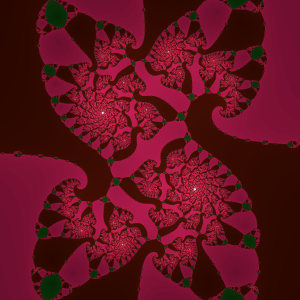On the G'MIC pipeline images are indivisible. Except through command line arguments, how blur proceeds is beyond anyone's ken. It admits no other interventions. Most times, such opacity is welcome; people have more than enough concerns without devils in details. But should details matter, then G'MIC's Mathematical Expressions goes to such depths. Mathematical expressions operate from within images — on the very bitmaps comprising images. G'MIC's pipeline process can invoke mathematical expressions only within certain settings, which set up their context. When invoked, they operate within the ambit of a complete programming language, one where rasters of any number of images can be directly accessed, along with metrics within the image environment itself.

Mathematical expressions may appear in three settings:

 1 Logic Flags: Mathematical expressions furnish logical flags for flow control commands. The return value is taken to be a boolean. See Example One. 2 Raster Processing: Mathematical expressions implement pel or pixel processors. The return value is taken to be a per-pel scalar or per-pixel vector; What the last statement returns establishes the processor type. Vector return values establish pixel processors; scalar return values establish pel processors. In any case, return values set the pel or pixel at the current location of the iteration. See Example Two. 3 Image Feature Substitution Expressions: For short: image directives: { , } The second argument of the default directive embeds a mathematical expression. The results of its evaluation may initialize pipeline variables or command arguments. See Example Three.

Within these settings, mathematical expressions are built up from sequences of statements separated by semicolons; the trailing semi-colon may be omitted; the aggregate constitutes the expression. The last statement connects the mathematical expression with its pipeline setting. The computed value of that last statement becomes the expression's return value and the one datum the pipeline process obtains from the expression. That return value's disposition follows from the setting: establishing a logical state, setting the value of a pel or pixel, or becoming the value to some key or the value of an argument.

Except for corner cases — perhaps an empty image stack — mathematical expressions operate with reference images. These establish many of the circumstances in which mathematical expressions operate, including a host of predefined variables conveying image metrics such as iv (variance) or ia (median value).
 1 The last item on the image list furnishes the reference for logical expressions. 2 The currently processing image is the reference for raster processors. 3 In image directives, { , }, A prefix integer, comma-separated from the math expression, selects the reference image via its list index. Without this prefix, the reference image defaults to the last image on the list. Name labels may appear instead of image indices, but the allied collection should contain only one image: a multi-image collection raises errors as it is impossible to determine which image is the reference.

An absent reference image is not an error. Math expressions just operate in curtailed environments; computations not dependent on image data still take place:
\$ gmic e '{cexp([0,47.265°])}'
[gmic]-0./ Start G'MIC interpreter.
0.67860847708958572,0.73450019388843812
[gmic]-0./ End G'MIC interpreter.
G'MIC works as a handy calculator — especially useful for those fraught moments when you simply must know the cosine and sine of 47.265°

Example One:
You want to base an output on overall image variance (iv):

reducevariance:
-skip \${1=5},\${2=5},\${3=1000},\${4=1}
nlevel,varcutoff,range,bstp=\${1-4}
-noise. \$nlevel%,2
-dilate_circ 3,2
-normalize. -\$range,\$range
-do
-blur. \$bstp,1
-while iv>\$varcutoff
——————
\$ gmic                                 \
reducevariance.gmic                 \
-input 300,300,1,3                  \
-name. canvas                       \
-reducevariance[canvas] 1,20,1000,1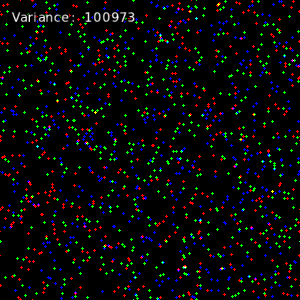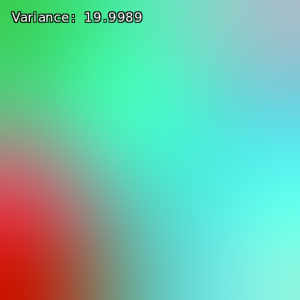Variance Mostly invariant variance
 1 A mathematical expression, iv>\$varcutoff, operating as a logical flag, asserts a do … while exit condition. Iterative blurring ceases when the current image's variance, the predefined math expression variable iv, is no longer greater than a supplied \$varcutoff level. 2 G'MIC's pipeline interpreter dereferences such keys as \$varcutoff before processing mathematical expressions. Here, the pipeline iterpreter first dereferences the key \$varcutoff and substitutes its value within the math expression, then turns the expression over to the mathematical expression parser. That parser does not "see" the string iv>\$varcutoff, but instead iv>81.25, presuming a user-supplied third argument of 81.25. G'MIC always dereferences pipeline variables — substituting keys by their values — before evaluating math expressions. By this, one may write pipeline variables in math expressions; after key⇒value substitutions, the mathematical expression parser consumes the value, not the key. See Substitution Cheats.

Example Two:
You want to produce an image reflecting the averaged, absolute differences of luminance between pixels and their immediate ±x-axis neighbors.
xdiff:
-luminance
-fill. (abs((j(-1,0)-i))+abs(i-j(1,0)))/2
-normalize. 0,255
——————
\$ gmic xdiff.gmic -input forest.png -verbose + +xdiff. -output. differed_forest.png
[gmic]-0./ Start G'MIC interpreter.
[gmic]-0./ Input custom command file 'xdiff.gmic' (1 new, total: 4572).
[gmic]-0./ Input file 'forest.png' at position 0 (1 image 768x768x1x3).
[gmic]-1./ Increment verbosity level (set to 2).
[gmic]-1./xdiff/ Compute luminance of image .
[gmic]-1./xdiff/ Fill image  with expression '(abs((j(-1,0)-i))+abs(i-j(1,0)))/2'.
[gmic]-1./xdiff/ Normalize image  in range [0,255], with constant-case ratio 0.
[gmic]-2./ Output image  as png file 'img/differed_forestflowers.png'.
[gmic]-2./ End G'MIC interpreter.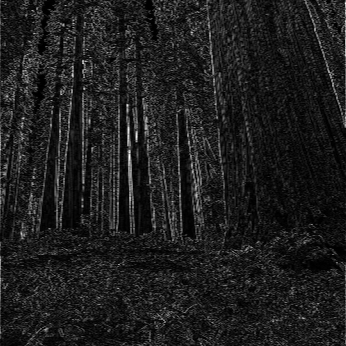Forest Differed Forest
 1. Commands input, fill and eval each may take pel or pixel processor arguments, these to define, change or evaluate images procedurally. a. If given, input repeatedly applies its fifth argument — a math expression — to each pel or pixel, part-and-parcel with image initialization. b. Similarly, fill alters bitmaps in accordance with its math expression argument, also applied to every pixel or pel. c. Like fill, eval also takes a math expression argument and iterates over the raster, but the last statement in the expresion does not automatically alter the bitmap. Such expressions operate for their side-effects instead. 2. The heart of xdiff is the fill command and its pel processor argument:                                                      (abs((j(-1,0)-i))+abs(i-j(1,0)))/2. Those new to math expressions may be forgiven if such seem Delphic: Oh Pythia! What does this mean? Are the Persians coming or not? (She just smiles). a. Pel processors iterate over the channel rasters themselves, one picture element (pel) at a time: a scalar. With the present example, the processor takes the average of the absolute values of two differences, these of center pels and their x-axis predecessors and successors. Such computations exhibits edge sensitivity. b. When, along the x-axis, the center pel is more-or-less equal to its predecessor and sucessor, the two differences are small; so follows the average of their absolutes. the image locality exhibits shallow gradients; the results exhibits only low luminance. c. On the other hand, differences vary greatly in localities of steep gradients; the results exhibit high luminance because the differences and their absolute averages are large.

It is worth emphasizing that the mathematical expression implementing this differencing pel processor executes once for every channel value (a.k.a. pel). So, a 768×768 image with red, green and blue channels induces 768×768×3=1,769,472 executions. Fashioning a vector-based final statement makes a pixel processor; this variant can be faster; the return value updates all image channels per each x, y location; only 768×768=589,824 executions occur.

In concert with shortcut pipeline commands, the common mathematical functions tend to terseness. For example, i() and j(), are pixel access operators. These are almost always present in raster processors as they directly query bitmaps. In this example, they take on the thirty-two bit float values of pels in each iteration location ( i(x,y,z,c) ), or ± x-axis offsets ( j(Δx,Δy,Δz,Δc) ). When the iteration completes, the pel processor has "filled" the selected image with a multitude of return pels, overwriting whatever was in place before.

Example Three:
You want to compute a value to initialize a pipeline variable and command arguments, these based on certain characteristics of the reference image. For that, use the default image directive; see Substitution Expressions.
1 vdiffmedian:
2   -name. original
3   oave={\$original,_ia}
4   +text_outline. "Average:\ "\$oave,3%,82%,7.5%,1,1,255,240,200
5   -name. {0,n}"_0"
6   -repeat 5
7     trial={\$>+1}
8     mset={5*\$trial}
9     +median[original] \$mset,\$oave
10     vdiff={_iv#\$original-iv}
11     -text_outline. "#\ "\$trial"\ Med:\ "\$mset,3%,82%,7.5%,1,1,255,240,200
12     -text_outline. "dvar:\ "\$vdiff,3%,90%,7.5%,1,1,255,240,200
13     -name. {0,n}"_"\$trial
14   -done
15   -display[^0]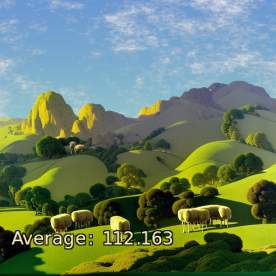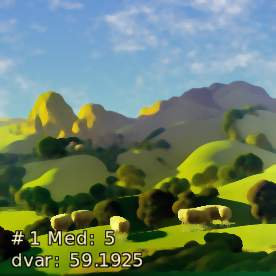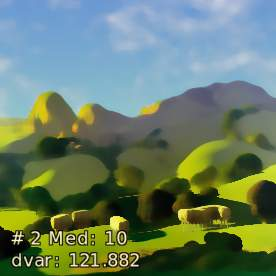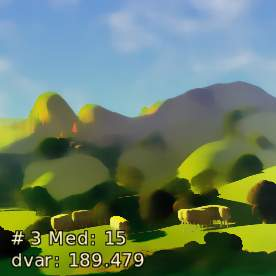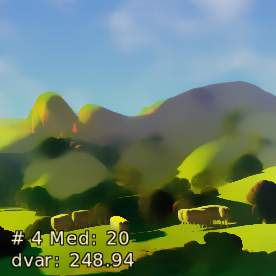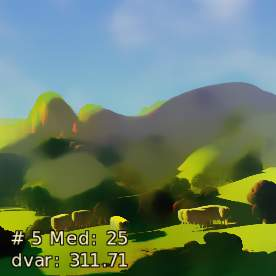vdiffmedian is a finger exercise that one might carry out to better understand the effect of pipeline commands and their arguments. Here, median lies on the examination table. Its first argument, radius, operates much like the corresponding blur argument; it sizes the blurring kernel — the neighborhood over which the command averages pel values. Larger radii yield greater spreads of one region into another. median 's second argument, threshold preserves gradients arising from local value changes that are greater than the specified argument. In this case, the average value of the original image is being harnessed to establish the threshold at which median holds edges. The overall effect can be dream-like. dvar, the difference between the image variance of the original and a median-blurred copy, is being investigated as a trial "dream-like" factor; as a current image is progressively blurred, its variance tends to drop. Consequently, the difference between the original variance and the current grows.

This finger exercise strives to grasp how median thresholding holds edges that are at least as pronounced as the average value of the original. Other benchmarks can be chosen and readers are welcome to devise any that suits a fancy. The heart of this finger exercise is the repeat ... done loop with a fixed number of steps. It steps the radius argument by increments of five. The exercise holds threshold steady as radius varies. Progressively larger radii give rise to progressively higher "dream-like" factors.

There are a number of image directives at play:
 1 Line 3: {\$original,_ia} is an image directive where a named collection, original specifies the reference image. More commonly, an explicit image list index may appear instead. The cited collection ( original ) should contain only one image, otherwise G'MIC can't determine what in a multi-member collection is to serve as a reference. In this case, only the selected image is in the collection so there is no ambiguity. The mathematical expression in this particular image feature is simply _ia. The leading underscore is a "round numeric display" operator, which truncates results to a limited number of significant digits. The mathematical expression consists of a single statement: _ia, the overall average value of the named image original. Necessarily the last statement in the expression, it sets the value to be associated with the key oave, used down-script (Line 9) to set the threshold argument. 2 Line  5: The image directive here, {0,n}, is not the default, which takes a mathematical expression, but one of the letter directive variants. Here, the single letter directive, n, identifies this as a request for the collection name in which the image could be enrolled. As with all image directives, the first argument is usually an image index; if omitted, then the last image on the list is implied. G'MIC replaces this directive with the collection name, if the image is enrolled in one, or the name of the image source that supplied the image, if there is one, or the default indicator [unnamed] if the image exists only on the stack. 3 Line  7: {\$>+1} is an image directive lacking an initial index argument; its reference image defaults to the last one on the image list. This matter is moot, however: the single statement entails no image-dependent feature or metric. As noted in Example One, above, G'MIC dereferences the key \$> substituting it for its value. This particular key is among those pre-defined substitutions reserved for repeat''…-done'' loop counts and substitutes for ascending loop counting. Its descending counterpart is \$<. This loop count, offset by one, becomes the trial count and, from the point of view of the math expression parser, the statement is a straightforward sum of two constants, the value associated with the key \$> and numeric 1. 4 Line  8: {5*\$trial} operates in a similar fashion to the image directive on the previous line: \$trial is the ones-based ascending loop count; this count, multiplied by five, becomes the current blurring radius: \$mset. The computation embodies the only statement in the expression; necessarily, it's the last and defines the value for key mset. 5 Line 10: {_iv#\$original-iv} takes the difference between two image metrics: the variance of the original image and the reference image. The postfix qualifier #\$original is the mechanism to reference any other image on the list. Commonly, an explicit image list index follows the hashtag # but the name of a collection, appears here. \$original. This use of a collection name may parse, so long as the collection original contains only one image. Collections of greater size gives rise to ambiguities; G'MIC will throw an error with multi-member collections.

Postscript
This Whitman Sampler of expressions furnish fairly short, pithy examples. Be that as it may, mathematical expressions can rise to meet fairly sophisticated aims — as the frontpiece demonstrates: a Newton-Raphson fractal generated from a pel processor. Download the  Newton-Raphson Generator  for its implementation. It is only a matter of degree, not kind, that separates the fractal generator from the one-liner illustrated in Example 2. At the end of the day, they are both pel processors, each operating in a raster processing context provided by fill. The following topics cover this traversal from the lower, simpler degrees to the more rarified realms:

 Basics Writing basic expressions, up to, but not including, conditional branching. This may be termed 'Old School Mathematical Expressions' of the sort that accompanied the early 1.x versions of G'MIC. Conditionals Adding logical branching to the basic mix. Scalars, Strings and Vectors A Deep dive on how bits persist in math expressions. Sharing Data With The Pipeline At computation's end, math expressions disappear completely — but messages can be put in bottles. Dynamic Arrays For when you don't know how much is enough. Raster Processing You may not even need a raster. Structured Drawing Ellipses and Polygons, Oh My! Macros Hide Repeating Chunks of Math Expressions With Short Hand. Threaded Expressions Walking and chewing gum at the same time.

Alas — ! The Mathematical Expression tutorials are still a work-in-progress (May 2023). Colored topics are in planning, outlining, writing, proofreading or revising stages — anywhere but here. When they arrive here, the corresponding link will go to black bold face. Stay tuned…

Updated: Sunday May 07 2023 16:50 UTC commit: a9d3c41965e12471c1e48a2a57a34c5d8fd3f62d
G'MIC - GREYC's Magic for Image Computing: A Full-Featured Open-Source Framework for Image Processing